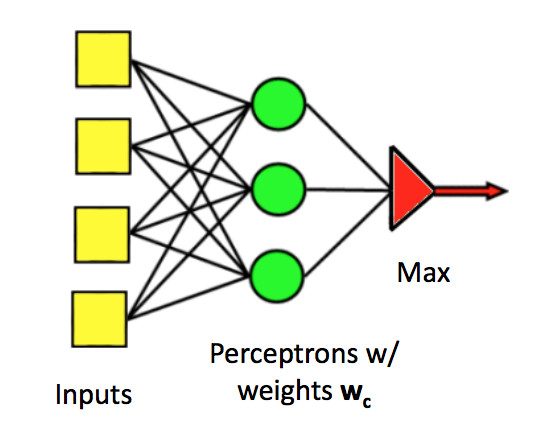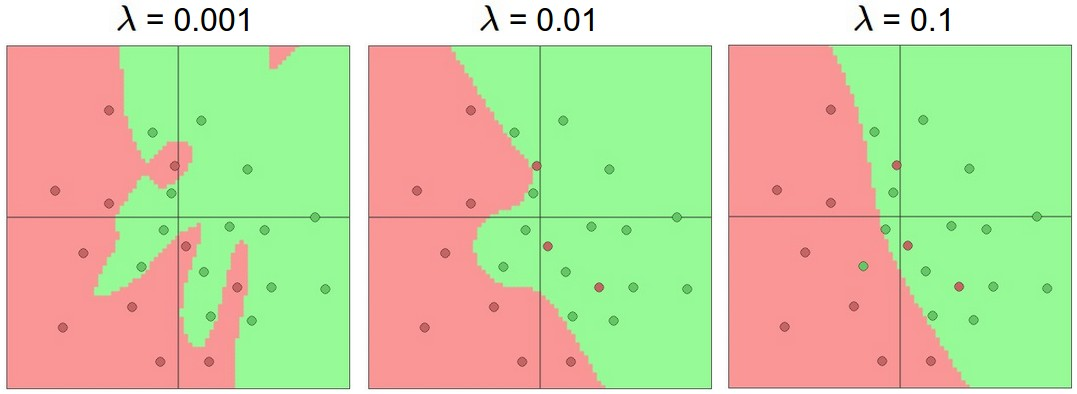CS 440/ECE 448
Margaret Fleck

## Linear Classifiers 4

We've seen how to define and train a single differentiable unit. It's useful to know about a couple more details before we move on to looking at multi-layer networks formed from these units.

## Softmax

Recall how we built a multi-class perceptron. We fed each input feature to all of the units. Then we joined their outputs together with an argmax to select the class with the highest output.(from Mark Hasegawa-Johnson, CS 440, Spring 2018)

We'd like to do something similar with differentiable units.

There is no (reasonable) way to produce a single output variable that takes discrete values (one per class). So we use a "one-hot" representation of the correct output. A one-hot representation is a vector with one element per class. The target class gets the value 1 and other classes get zero. E.g. if we have 8 classes and we want to specify the third one, our vector will look like:

[0, 0, 1, 0, 0, 0, 0, 0]


One-hot doesn't scale well to large sets of classes, but works well for medium-sized collections.

Now, suppose we have a sequence of classifier outputs, one per class. $$v_1,\ldots,v_n$$. We often have little control over the range of numbers in the $$v_i$$ values. Some might even be negative. We'd like to map these outputs to a one-hot vector. Softmax is a differentiable function that approximates this discrete behavior. It's best thought of as a version of argmax.

Specifically, softmax maps each $$v_i$$ to $$\displaystyle \frac{e^{v_i}}{\sum_i e^{v_i}}$$. The exponentiation accentuates the contrast between the largest value and the others, and forces all the values to be positive. The denominator normalizes all the values into the range [0,1], so they look like probabilities (for folks who want to see them as probabilities).

## Regularization

Any type of model fitting is subject to possible overfitting. When this seems to be creating problems, a useful trick is "regularization." Suppose that our set of training data is T. Then, rather than minimizing loss(model,T), we minimize

loss(model,T) + $$\lambda$$ * complexity(model)

The right way to measure model complexity depends somewhat on the task. A simple method for a linear classifier would be $$\sum_i (w_i)^2$$, where the $$w_i$$ are the classifier's weights. Recall that squaring makes the L2 norm sensitive to outlier (aka wrong) input values. In this case, this behavior is a feature: this measure of model complexity will pay strong attention to unusually large weights and make the classifier less likely to use them. Linguistic methods based on "minimumum description length" are using a variant of the same idea.

$$\lambda$$ is a constant that balances the desire to fit the data with the desire to produce a simple model. This is yet another parameter that would need to be tuned experimentally using our development data. The picture below illustrates how the output of a neural net classifier becomes simpler as $$\lambda$$ is increased. (The neural net in this example is more complex, with hidden units.)from Andrej Karpathy course notes